Atomic Model of the Solar System Putting into Evidence a Tenth Celestial Object Coupled To Pluto | OMICS International
Journal of Astrophysics & Aerospace Technology

# Atomic Model of the Solar System Putting into Evidence a Tenth Celestial Object Coupled To Pluto

Sakho I*

Department of Physics, UFR of Sciences and Technologies, University Assane Seck of Ziguinchor, Ziguinchor, Senegal

*Corresponding Author:
Sakho I
Department of Physics
UFR of Sciences and Technologies
University Assane Seck of Ziguinchor
Ziguinchor, Senegal
Tel: 0022177 500 21 65
E-mail: [email protected]

Received date: November 03, 2016; Accepted date: November 14, 2016; Published date: February 14, 2017

Citation: Sakho I (2017) Atomic Model of the Solar System Putting into Evidence a Tenth Celestial Object Coupled To Pluto. J Astrophys Aerospace Technol 4:135. doi:10.4172/2329-6542.1000135

Copyright: © 2017 Sakho I. This is an open-access article distributed under the terms of the Creative Commons Attribution License, which permits unrestricted use, distribution, and reproduction in any medium, provided the original author and source are credited.

Visit for more related articles at Journal of Astrophysics & Aerospace Technology

#### Abstract

Description of a new model of the Solar System is given in this paper. Analogously to the planetary atomic model of Rutherford, we describe an Atomic Model of the Solar System (AMSS) in which the planets are coupled like electrons in atoms. The AMSS presented put into evidence the existence of a tenth celestial object (nicknamed here SENEG) that might be the centuries-long searched tenth planet or classified in the category of dwarf planets. Since the much controverted Prague (IAU0603 2006) statement, astronomers actively search beyond Neptune a probable ninth planet. The AMSS model gives in the viewpoint of mathematics, the criterion satisfied by a planet belonging to the Solar System. The AMSS indicates that, the stability of the Solar System is only compatible with a configuration containing ten planets and that SENEG orbits the Sun at the distance of 11,208,000,000 km. From the AMSS model, it is mathematically shown that both 2003 VB12 (Sedna) and 2003 UB313 (Xena) have not the status of planets and they may be classified in the category of dwarf planets. Discussion about how the AMSS model may play a prominent role in the implementation of the quantum theory of freedmons is also made.

#### Keywords

Solar system; Dwarf planets; Atomic model of the solar system; Freedmon

#### Introduction

In the absence of an exact theory on the Solar System, one can only perform planetary models based on a certain number of simplified hypotheses. Each of these approximate models gives only a partial information of the granularly structure of the Solar System. In the past, nine planets (from Mercury to Pluto) have been discovered as belonging to the Solar System. Formerly, two new ‘’planets’’ referred as 2003VB12  and 2003UB313 [1-3] have been discovered and considered as belonging to the Solar. During the 26th general assembly of International Astronomical Union (IAU) which has took place on the 14 and 25 of August 2006 at Prague, Pluto has been declined of its status of planet . Since the much-controverted Prague (IAU0603 2006) statement, astronomers actively search beyond Neptune a probable ninth planet . Obviously, the number of planets of the Solar System is not infinite. Subsequently, it would be very challenging in the view point of mathematics, to establish the criterion satisfied by a planet belonging to the Solar System. In addition, the stability of the Solar System depends on its number of planets and on how these planets are configured in the planetary cloud of the Sun. The centrifugal force due to the orbital motion of a planet may not be sufficient to counterbalance the attractive gravitational force from the Sun. That means, the stability of the orbit of a given planet of the Solar System is probably due to additional strong attractive planet-planet interaction. This assumption suggests to consider a configuration in which the planets are coupled like electrons in atoms. So attractive interaction between two coupled planets contributes to their stability and to that of the whole Solar System. In the past, Rutherford managed to explain the stability of the atomic systems by proposing a planetary model of the atom. Adopting an opposite procedure to that of Rutherford, we describe an atomic model of the Solar System (AMSS) putting into evidence the existence of a tenth celestial object (that may be a planet or a dwarf planet). We assume that, at a certain moment of its formation, the Solar System captured a tenth celestial object for its stability. In the framework of the AMSS model, the planets orbiting the Sun are then coupled. On the other hand, it’s generally believed that, the only sure method to identify the tenth planet is to know its exact situation in the firmament and then to discover it at the indicated place . The following study is in this direction. In section 2 we present our atomic model of the Solar System. In section 3 the presentation and the discussion of our results are made. Comparison is also done with available astronomical observations.

#### Theory

Some similarities between the Solar System and the atomic systems

In the following study, we adopt the model of the independent particles that disregards planet-planet interaction and electronelectron interaction. Then, for a given system (astronomic or atomic), direct interaction of the particle (planet or electron) with the center of the system (Sun or Nucleus) is considered. We use also the model of the Copernican Solar System and the Bohr’s atomic model in the approximation of the circular orbit of the particles (planets or electrons respectively). In Table 1, some elements of comparison are displayed. These elements listed in Table 1 show without doubt that there is no contradiction between the macrocosm and the microcosm. How then one can explain discrepancies between the Solar System and the atomic systems in view of the analogical elements quoted in the seventeenth and eighteenth lines of Table 1? Nothing could logically explain that. Then we assume that, like electrons in atoms, the planets in the Solar System are coupled. As a result, Pluto might be coupled to a tenth celestial object (planet or dwarf planet).

Elements of analogy Solar System Atomic system
Center of rotation Sun Nucleus
Own motion of the center Turning around its own axis
(Spinning)
Turning around its own axis
(Spinning)
Satellites objects Planets Electrons
Nature of the motion of the satellites objects with respect to the center Each planet turns around
the Sun
Each electron turns around
the Nucleus
Own motion of the Satellites objects Each planet turns around�? its own axis�? (spinning) Each electron turns around its own axis (spinning)
Nature of the radiation emitted inside de center Emission of gamma radiation by the Sun Emission of gamma radiation by the Nucleus
Secondary emissions Emission of X-rays, UV, and IR radiations in the atmospheric planetarium�? layers Emission of X-rays, UV, and IR radiations in the electronic atomic�? layers
Type of structure Lacunar
99.87% of the mass of the Solar System is concentered in the Sun
Lacunar
99.98% of the mass of the atomic system is concentered in the Nucleus
Nature of the interaction force between the center and the satellites objects Central force in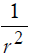Central force inTotal energy of the system{Center-Satellite}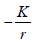K  a constant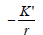K’ a constant
Vector particle in the viewpoint of the new interpretation of the fourth fundamental interactions Graviton Carrying the gravitational
force
Gamma (photon) Carrying the electromagnetic force
Mass of the vector particle 0 0
Range of the interaction Infinite Infinite
Ve locity of the vector particle c
Velocity of light
c
Velocity of light
Statistics of the vector particle Bose-Einstein Bose-Einstein
Configuration of the satellites objects ? Electrons are coupled in quantum cells
Intrinsic property of the satellites objects etc. ? Each electron has a spin angular momentum equal to 1/2

Table 1: Some resemblances between the Solar System and an atomic system of many electrons. The exact resemblances from line 1 to line 15 indicate that, the planets may be coupled like electrons in atoms and have then probably quantum angular momentum of spin.

Simple laws satisfied by the coupled planets

In Table 2, we have listed some well known characteristics of the planets of the Solar System. Using these characteristics let’s us calculate the following ratios:In these ratios, n denotes the rank of the inner coupled planet with respect to the Sun, V, d and T represent respectively the orbital speed, the average distance to the Sun and the period of the orbital motion of the considered planet. By use of the values quoted in Table 2, we find:

Characteristics
Planet Average orbital speed
(km/s)
Average distance
Planet-Sun (× 109 m)
Period of the orbital motion (× 106 s)
Mercury 47.84 57.84 7.60
Venus 35.00 108.14 19.4
Earth 29.77 149.5 31.6
Mars 24.11 227.8 59.4
Jupiter 13.05 777.8 375
Saturn 9.64 1426 930
Uranus 6.80 2868 2660
Neptune 5.43 4494 5200
Pluto 4.73 5908 7820

Table 2: Some characteristics of the nine planets of the solar system.

•For the ratios of the orbital speed: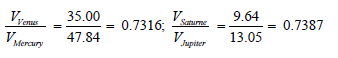(1)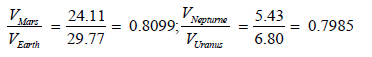(2)

• For the cubic ratios of the average distance with respect to the Sun (Table 3):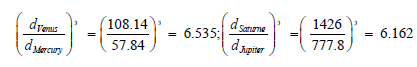(3)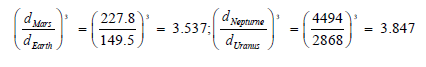(4)

• For the square ratios of the orbital motion period: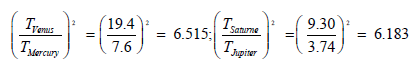(5)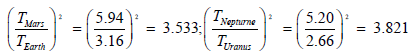(6)

Considering the preceding results, we deduce the simple following laws.

First law: Law of variation of the ratio of the average orbital speed of the coupled planets

For a couple of planets considered, if n denotes the rank of the inner planet of the couple with respect to the Sun, the ratios of their average orbital speed satisfy the condition,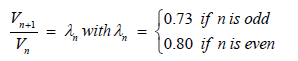(7)

Second law: Law of variation of the ratio of the average distance to the Sun of the coupled planets

For a couple of planets considered, if n denotes the rank of the inner planet of the couple with respect to the Sun, the ratios of their average distance to the Sun satisfy the condition,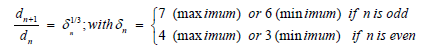(8)

Third law: Law of variation of the ratio of the average period of the orbital motion of the coupled planets

For a couple of planets considered, if n denotes the rank of the inner planet of the couple with respect to the Sun, the ratios of their average period of the orbital motion satisfy the condition (Table 3),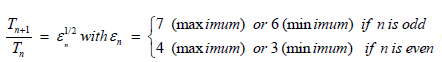(8)

Characteristics
Couple Average orbital speed
(km/s)
Average distance
Planet-Sun (× 109 m)
Period of the orbital motion (× 106 s)
Mercury 47.84 57.84 7.60
Venus 35.00 108.14 19.4
Earth 29.77 149.5 31.6
Mars 24.11 227.8 59.4
Jupiter 13.05 777.8 375
Saturn 9.64 1426 930
Uranus 6.80 2868 2660
Neptune 5.43 4494 5200
Pluto 4.73 5908 7820
Seneg 3.45 11208 20520

Table 3: Average orbital speed, average distance with respect to the Sun and average of the orbital motion period of the coupled planets of the solar system.

#### Results and Discussion

According to the AMSS model, the tenth celestial object nicknamed SENEG is coupled to Pluto.

Average orbital speed of the tenth celestial object

Using the first law (7), we find,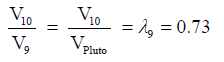(9)

As VPluto = 4.73 km/s, equation (9) gives for SENEG,

V10 = 3.45 km/s

Framing of the average distance of the tenth celestial with respect to the Sun

As it can be noticed in the formulation of the second law (8), only a framing of the average distance of the tenth celestial object with respect to the Sun can be rigorously established. Then according to this law, we find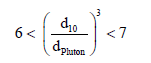(11)

As dPluto = 5908 109 m, double inequality (11) gives,

10735 109m < d10 < 11302 109m (12)

In astronomical unity 1AU = 149.5 109m, the result (12) becomes,

1.81 AU < d10< 75.60 AU (13)

But as the average orbital speed V and the average distance d of a planet are linked by the well known formula: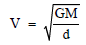(14)

where G denotes the gravitation constant and M the mass of the Sun.

In the basis of G = 6.67 10-11 SI; M = 2 1030 kg and V10 = 3.45 km/s, equation (14) gives,

d10 = 11 208 000 000 km (15)

This result agrees well with the condition (12).

Framing of the average period of the orbital motion of the tenth celestial object

By use of the third law we find,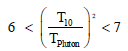(16)

As TPluto = 7.82 109 s, double inequality (16) gives,

9.15 109 s < T10 < 20.69 109 s

On the other hand, d and T are linked by the third law of Kepler,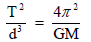(18)

Using the value (15) of d10, equation (18) gives for T10 the result,

T10 = 20.52 109 m (19)

This result agrees well also with the condition (17).

For comparison, let us remind former astronomical observations. In the past, a group of five comets have been discovered and their aphelia are about 50,800,000,000 km of the Sun. This localises them around the orbit of Pluto. In addition, for sixteen identified comets, the aphelia is situated to more than 11,000,000,000 kilometres of the Sun [6,7]. As most of things relative to the Solar System, the progression of the Bode’s law [8,9] (Table 4) considered especially as an amusing curiosity supposed that the sixteen identified comets which the aphelia is at 11,000,000,000 kilometres of the Sun are dominated by a tenth planet who follows an orbit with an average distance to the Sun equals to this aphelia . The preceding astronomical observations agree well with our theoretical result (15). This indicates that, the tenth celestial object might be researched among the sixteen formerly identified comets which the aphelia is at more than 11,000,000,000 kilometres of the Sun (Table 4).

Characteristics
Name of the celestial object Average distance
Planet-Sun
Average distance Planet-Sun
According to the Bode’ law*

Mercury

0.39 0.4

Venus

0.72 0.7

Earth

1.0 1.0

Mars

1.52 1.6

Asteroids

2.65 (approx.) 2.8

Jupiter

5.2 5.2

Saturn

9.64 10

Uranus

19.19 19.6

Neptune

30.05 38.8

Pluto

39.52 77.2

Table 4: Comparison of the distance of the ninth planets of the solar system with the predictions of the Bode’s law.

Consequences from AMSS

Is 2003 VB12 (Sedna) or 2003 UB313 (Xena) the hypothetical tenth planet?: In the year 2003, astronomical observations have instituted debates between two planets supposed to be the tenth planet. Astronomers at California Institute of Technology, manage then to identify by use of Samuel Oschin Telescope (Palomar mount) two planets assumed to belong to the Solar System. These planets were baptized 2003VB12 (Sedna) and 2003UB313 (Xena) [3,4,7]. The distances of these two planets with respect to the Sun are respectively equal to 91 UA and 97 UA [3,4,7]. Let’s us calculate for each of them the ratio (d10/dPluto)3. We find respectively: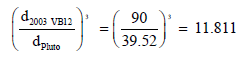(20)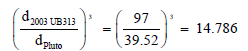(21)

These results show that the condition (11) is not satisfied. Then both 2003 VB12 and 2003 UB313 cannot have the status of a planet. This result matches well with what is accepted for their celestial bodies classified in the category of Ceres in motion between Mars and Jupiter and are then considered as dwarf planets . Let us know turn on debating the Prague statement considering the Solar System as composed of eight planets, both Pluto and 2003 UB313 being dwarf planets category . According to the new definition, two conditions must be satisfied for an object to be called a "planet." Firstly, the object must be in orbit around a star, while not being itself a star. Secondly, the object must be large enough (or more technically correct, massive enough) for its own gravity to pull it into a nearly spherical shape. The shape of objects with mass above 5 ×1020 kg and diameter greater than 800 km would normally be determined by self-gravity, but all borderline cases would have to be established by observations . Then, it is on the basis of the new definition of a planet that Pluto has been dethroned since 2006. As the planetary model of the atom is correct, the atomic model of the Solar System merits attracting attention by the only fact that it will help physicists and astronomers to find the way for conciliating the macrocosm and the microcosm [6,7]. The three laws established above indicate that the planets are probably coupled and then can be considered as the main credit of the validity of our AMSS. As both 2003 VB12 and 2003 UB313 are not coupled to Pluto, legitimately SENEG might be the celestial body of the Solar System coupled to Pluto and they might have the same status (planet or dwarf planet). This means that, if SENEG is a planet, the status of Pluto will be restored as a planet. Subsequently, the discovery of SENEG by photography using for instance the Samuel Oschin Telescope at Palomar Observatory, will involve inevitably a reevaluation of the Prague 2006 statement as far as the status of Pluto is concerned [9,10].

About the quantum theory of freedmon: As emphasize by Ginzburg [11,12], “For a distant exterior observer, the whole world can appear like a small particle, a freedmon. In the framework of the classical theory, the mass, the charge and the other parameters (the spin) of the freedmon have not been yet determined and the quantum theory of freedmons is not yet established. However, one can hope that, in the quantum theory, freedmons possess parameters of the elementary particles. At present hour, it is a fantastic eventuality, a hope, a dream. But it merits attracting attention by the only fact that it will help physicists to find the way for conciliating the contradictions (the macrocosm and the microcosm)”. According to our atomic model of the Solar System, the planets are coupled and might have quantum angular momentum of spin like electrons. The AMSS model presented suggests considering each planet as a freedmon of spin ½. In fact, the concept of spin is borrowed to the word spinning which means turning around its own axis like a classical spinning top. As all planets are spinning, one can well assume legitimately that they are characterised by an angular momentum of spin. If the planetarium spin is equal to that of the electron (1/2), this will be a great path toward the way leading to the unification of the macrocosm and the microcosm. We hope that our atomic model of the Solar System will greatly help in the development of the quantum theory of freedmons. Previously, we have demonstrated the possibilities to understand the organization and the evolution of astronomical systems by dealing with analogy between the properties of elementary particles and astrophysical objects [10-12]. The quantum theory of freedmons might be the more exciting astrophysical topic in the forthcoming decade.

#### Conclusion

With a simple atomic model of the Solar System, we have putted into evidence the existence of a tenth “planet” for the stability of the Solar System. The model presented in this work permits the calculation of the distance of the tenth planet with respect to the Sun which is the principal characteristic for identifying it in the firmament. The present study has also the merit to fix the conditions to be satisfied by a planet belonging to the Solar System. This point is extremely important as it is obvious that the Solar System cannot contain infinite celestial objects. On the other hand, the similarities shown in this paper through Table 1 between atomic configurations and the Solar System indicate that our atomic model of the Solar System may play an important role in the implementation of the quantum theory of freedmons. We name temporary SENEG as the tenth celestial object coupled to Pluto. In August 2006, the IUA stated that, a planet of the Solar System is a celestial body which at least is in orbit around the Sun and has sufficient mass to assume hydrostatic equilibrium. As the mass of SENEG is not known for the time being, it is not possible to state to its status of a planet or a dwarf planet.

#### References

Select your language of interest to view the total content in your interested language

### Article Usage

• Total views: 3047
• [From(publication date):
June-2017 - Jan 21, 2020]
• Breakdown by view type
• HTML page views : 2913Can't read the image? click here to refresh